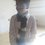# Science wiz! Please help

I was studying $\text{Thermal Expansion}$ from H.C. Verma - Concepts of Physics 2 (if you have the book, page 6 - topic 23.10). If you don't have the book, this is the concerned matter.

Note: If you know this stuff, you can jump to the end.

Consider a rod at temperature $T$ and suppose its length at this temperature is $L$. As the temperature is changed to $T+\Delta T$, the length is changed to $L+\Delta L$. We define average coefficient of linear expansion in the temperature range $\Delta T$ as $\overline{\alpha}=\frac{1}{L}\frac{\Delta L}{\Delta T}$ The coefficient of linear expansion at temperature $T$ is limit of average coefficient as $\Delta T\rightarrow0$, i.e., $\alpha=\displaystyle\lim_{\Delta T\rightarrow0}\frac{1}{L}\frac{\Delta L}{\Delta T}=\frac{1}{L}\frac{dL}{dT}$ Suppose the length of a rod is $L_{0}$ at $0^{\circ}C$ and $L_{\theta}$ at temperature $\theta$ measured in Celsius. If $\alpha$ is small and constant over the given temperature interval, $\alpha=\frac{L_{\theta}-L_{0}}{L_{0}\theta}$ or $L_{\theta}=L_{0}(1+\alpha\theta)$ The coefficient of volume expansion $\gamma$ is defined in a similar way. If $V$ is the volume of a body at temperature $T$, the coefficient of volume expansion at temperature $T$ is $\gamma=\frac{1}{V}\frac{dV}{dT}$ It is also known as coefficient of cubical expansion.

If $V_{0}$ and $V_{\theta}$ denote the volumes at $0^{\circ}C$ and $\theta$ (measured in Celsius) respectively and $\gamma$ is small and constant over the given temperature range, we have $V_{\theta}=V_{0}(1+\gamma\theta)$

It is easy to show that $\gamma=3\alpha$.

My question is: How do you prove that $\gamma=3\alpha$?

Note for those who jumped: $\gamma$ is the coefficient of cubical expansion, and $\alpha$ is the coefficient of linear expansion.Note by Omkar Kulkarni
6 years, 1 month ago

This discussion board is a place to discuss our Daily Challenges and the math and science related to those challenges. Explanations are more than just a solution — they should explain the steps and thinking strategies that you used to obtain the solution. Comments should further the discussion of math and science.

When posting on Brilliant:

• Use the emojis to react to an explanation, whether you're congratulating a job well done , or just really confused .
• Ask specific questions about the challenge or the steps in somebody's explanation. Well-posed questions can add a lot to the discussion, but posting "I don't understand!" doesn't help anyone.
• Try to contribute something new to the discussion, whether it is an extension, generalization or other idea related to the challenge.
• Stay on topic — we're all here to learn more about math and science, not to hear about your favorite get-rich-quick scheme or current world events.

MarkdownAppears as
*italics* or _italics_ italics
**bold** or __bold__ bold
- bulleted- list
• bulleted
• list
1. numbered2. list
1. numbered
2. list
Note: you must add a full line of space before and after lists for them to show up correctly
paragraph 1paragraph 2

paragraph 1

paragraph 2

[example link](https://brilliant.org)example link
> This is a quote
This is a quote
    # I indented these lines
# 4 spaces, and now they show
# up as a code block.

print "hello world"
# I indented these lines
# 4 spaces, and now they show
# up as a code block.

print "hello world"
MathAppears as
Remember to wrap math in $$ ... $$ or $ ... $ to ensure proper formatting.
2 \times 3 $2 \times 3$
2^{34} $2^{34}$
a_{i-1} $a_{i-1}$
\frac{2}{3} $\frac{2}{3}$
\sqrt{2} $\sqrt{2}$
\sum_{i=1}^3 $\sum_{i=1}^3$
\sin \theta $\sin \theta$
\boxed{123} $\boxed{123}$

## Comments

Sort by:

Top Newest

We consider a cube of metal with each side equal to L.

If we heat it up, the length of each side will change from L to (L+ΔL), where ΔL = αLΔT.

In other words, the new length is:

L′ = L + αLΔT = L(1 + αΔT)

So the new volume is:

Vo′ = (L′)^3 = [L(1 + αΔT)]^3 = (L^3)(1 + 3αΔT + 3(αΔT)² + (αΔT)^3) = Vo(1 + 3αΔT + 3(αΔT)² + (αΔT)^3)

Now we make an approximation. Since αΔT is quite small compared to 1, we can safely say that the "3(αΔT)²" term and the "(αΔT)^3" term are negligible compared to the "3αΔT" term. Therefore, to a good approximation,

Vo′ = Vo(1 + 3αΔT)

But we already know, from the definition of γ, that:

Vo′ = Vo(1 + γΔT)

So this means that γ = 3α.

I could have written the solution in Latex but I am short on time. (NTSE upcoming)

- 6 years, 1 month ago

Log in to reply

Ohh okay! Makes sense now :) Thanks! All the best for NTSE.

- 6 years, 1 month ago

Log in to reply

Wait did you mean $\overline{\alpha}$ instead of $\alpha$?

- 6 years, 1 month ago

Log in to reply

Y=3a

- 6 years ago

Log in to reply

What?

- 6 years ago

Log in to reply

×

Problem Loading...

Note Loading...

Set Loading...